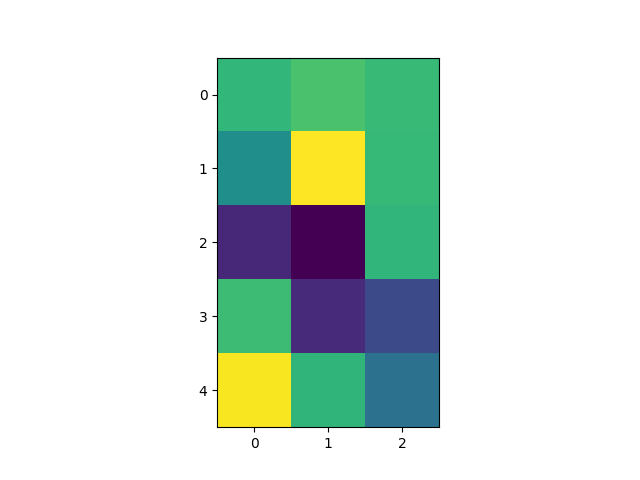# Modifying the coordinate formatter¶

Modify the coordinate formatter to report the image "z" value of the nearest pixel given x and y. This functionality is built in by default, but it is still useful to show how to customize the format_coord function.

import numpy as np
import matplotlib.pyplot as plt

# Fixing random state for reproducibility
np.random.seed(19680801)

X = 10*np.random.rand(5, 3)

fig, ax = plt.subplots()
ax.imshow(X)

numrows, numcols = X.shape

def format_coord(x, y):
col = int(x + 0.5)
row = int(y + 0.5)
if 0 <= col < numcols and 0 <= row < numrows:
z = X[row, col]
return 'x=%1.4f, y=%1.4f, z=%1.4f' % (x, y, z)
else:
return 'x=%1.4f, y=%1.4f' % (x, y)

ax.format_coord = format_coord
plt.show()References

The use of the following functions, methods, classes and modules is shown in this example:

Keywords: matplotlib code example, codex, python plot, pyplot Gallery generated by Sphinx-Gallery